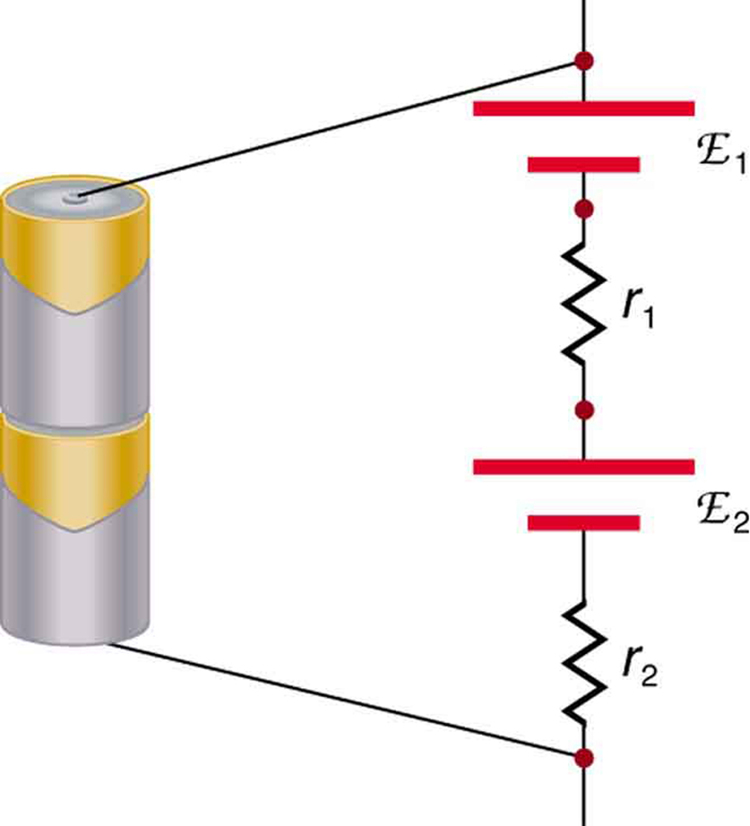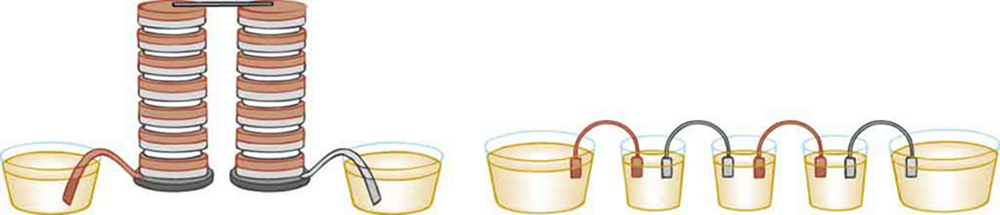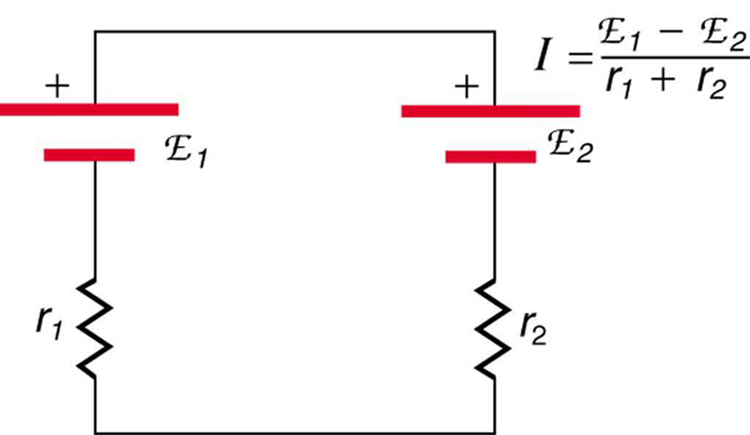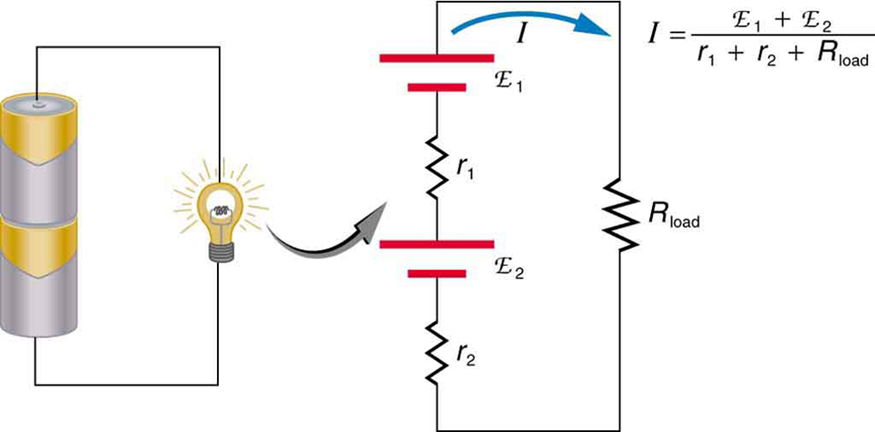# 21.2 Electromotive force: terminal voltage  (Page 5/12)

 Page 5 / 12

But if the cells oppose one another, such as when one is put into an appliance backward, the total emf is less, since it is the algebraic sum of the individual emfs.

A battery is a multiple connection of voltaic cells, as shown in [link] . The disadvantage of series connections of cells is that their internal resistances add. One of the authors once owned a 1957 MGA that had two 6-V batteries in series, rather than a single 12-V battery. This arrangement produced a large internal resistance that caused him many problems in starting the engine.A series connection of two voltage sources. The emfs (each labeled with a script E) and internal resistances add, giving a total emf of emf 1 + emf 2 size 12{"emf" rSub { size 8{1} } +"emf" rSub { size 8{2} } } {} and a total internal resistance of r 1 + r 2 size 12{r rSub { size 8{1} } +r rSub { size 8{2} } } {} .Batteries are multiple connections of individual cells, as shown in this modern rendition of an old print. Single cells, such as AA or C cells, are commonly called batteries, although this is technically incorrect.

If the series connection of two voltage sources is made into a complete circuit with the emfs in opposition, then a current of magnitude $I=\frac{\left({\text{emf}}_{1}–{\text{emf}}_{2}\right)}{{r}_{1}+\phantom{\rule{0.25em}{0ex}}{r}_{2}}$ flows. See [link] , for example, which shows a circuit exactly analogous to the battery charger discussed above. If two voltage sources in series with emfs in the same sense are connected to a load ${R}_{\text{load}}$ , as in [link] , then $I=\frac{\left({\text{emf}}_{1}+\phantom{\rule{0.25em}{0ex}}{\text{emf}}_{2}\right)}{{r}_{1}+\phantom{\rule{0.25em}{0ex}}{r}_{2}+{R}_{\text{load}}}$ flows.These two voltage sources are connected in series with their emfs in opposition. Current flows in the direction of the greater emf and is limited to I = emf 1 − emf 2 r 1 + r 2 size 12{I= { { left ("emf" rSub { size 8{1} } - "emf" rSub { size 8{2} } right )} over {r rSub { size 8{1} } +r rSub { size 8{2} } } } } {} by the sum of the internal resistances. (Note that each emf is represented by script E in the figure.) A battery charger connected to a battery is an example of such a connection. The charger must have a larger emf than the battery to reverse current through it.This schematic represents a flashlight with two cells (voltage sources) and a single bulb (load resistance) in series. The current that flows is I = emf 1 + emf 2 r 1 + r 2 + R load size 12{I= { { left ("emf" rSub { size 8{1} } - "emf" rSub { size 8{2} } right )} over {r rSub { size 8{1} } +r rSub { size 8{2} } +R rSub { size 8{"load"} } } } } {} . (Note that each emf is represented by script E in the figure.)

## Take-home experiment: flashlight batteries

Find a flashlight that uses several batteries and find new and old batteries. Based on the discussions in this module, predict the brightness of the flashlight when different combinations of batteries are used. Do your predictions match what you observe? Now place new batteries in the flashlight and leave the flashlight switched on for several hours. Is the flashlight still quite bright? Do the same with the old batteries. Is the flashlight as bright when left on for the same length of time with old and new batteries? What does this say for the case when you are limited in the number of available new batteries?

[link] shows two voltage sources with identical emfs in parallel and connected to a load resistance. In this simple case, the total emf is the same as the individual emfs. But the total internal resistance is reduced, since the internal resistances are in parallel. The parallel connection thus can produce a larger current.

does the force in a system result in the energy transfer?
full meaning of GPS system
how to prove that Newton's law of universal gravitation F = GmM ______ R²
sir dose it apply to the human system
prove that the centrimental force Fc= M1V² _________ r
prove that centripetal force Fc = MV² ______ r
Kaka
how lesers can transmit information
griffts bridge derivative
below me
please explain; when a glass rod is rubbed with silk, it becomes positive and the silk becomes negative- yet both attracts dust. does dust have third types of charge that is attracted to both positive and negative
what is a conductor
Timothy
hello
Timothy
below me
why below you
Timothy
no....I said below me ...... nothing below .....ok?
dust particles contains both positive and negative charge particles
Mbutene
corona charge can verify
Stephen
when pressure increases the temperature remain what?
remains the temperature
betuel
what is frequency
define precision briefly
CT scanners do not detect details smaller than about 0.5 mm. Is this limitation due to the wavelength of x rays? Explain.
hope this helps
what's critical angle
The Critical Angle Derivation So the critical angle is defined as the angle of incidence that provides an angle of refraction of 90-degrees. Make particular note that the critical angle is an angle of incidence value. For the water-air boundary, the critical angle is 48.6-degrees.
okay whatever
Chidalu
pls who can give the definition of relative density?
Temiloluwa
the ratio of the density of a substance to the density of a standard, usually water for a liquid or solid, and air for a gas.
Chidalu
What is momentum
mass ×velocity
Chidalu
it is the product of mass ×velocity of an object
Chidalu
how do I highlight a sentence]p? I select the sentence but get options like copy or web search but no highlight. tks. src
then you can edit your work anyway you wantByByBy OpenStaxBy Madison ChristianBy Christine ZeelieBy CB BiernByBy OpenStaxBy OpenStaxBy Jessica CollettBy Mary MateraBy Donyea Sweets